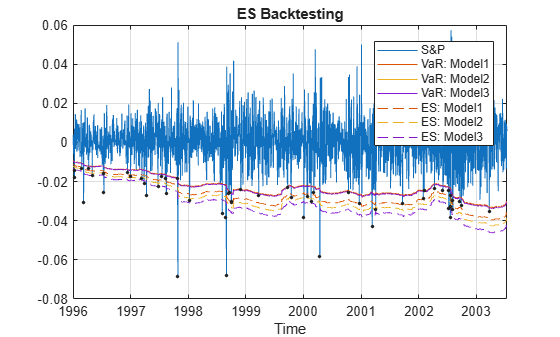# Expected Shortfall (ES) Backtesting Workflow with No Model Distribution Information

This example shows an expected shortfall (ES) backtesting workflow and the use of ES backtesting tools. The `esbacktest` class supports two tests -- unconditional normal and unconditional t -- which are based on Acerbi-Szekely's unconditional test statistic (also known as the Acerbi-Szekely second test). These tests use presimulated critical values for the unconditional test statistic, with an assumption of normal distribution for the normal case and a t distribution with 3 degrees of freedom for the t case.

### Step 1. Load the ES backtesting data.

Use the `ESBacktestData.mat` file to load the data into the workspace. This example works with the `Returns` numeric array. This array represents the equity returns, `VaRModel1`, `VaRModel2`, and `VaRModel3`, and the corresponding VaR data at 97.5% confidence levels, generated with three different models. The expected shortfall data is contained in `ESModel1`, `ESModel2`, and `ESModel3`. The three model distributions used to generate the expected shortfall data in this example are normal (model 1), t with 10 degrees of freedom (model 2), and t with 5 degrees of freedom (model 3). However, this distribution information is not needed in this example because the `esbacktest` object does not require it.

```load('ESBacktestData') whos```
``` Name Size Bytes Class Attributes Data 1966x13 223946 timetable Dates 1966x1 15728 datetime ESModel1 1966x1 15728 double ESModel2 1966x1 15728 double ESModel3 1966x1 15728 double Returns 1966x1 15728 double VaRLevel 1x1 8 double VaRModel1 1966x1 15728 double VaRModel2 1966x1 15728 double VaRModel3 1966x1 15728 double ```

### Step 2. Generate an ES backtesting plot.

Use the `plot` function to visualize the ES backtesting data. This type of visualization is a common first step when performing an ES backtesting analysis. For illustration purposes only, visualize the returns, together with VaR and ES, for a particular model.

The resulting plot shows some large violations in 1997, 1998, and 2000. The violations in 1996 look smaller in absolute terms, however relative to the volatility of that period, those violations are also significant. For the unconditional test, the magnitude of the violations and the number of violations make a difference, because the test statistic averages over the expected number of failures. If the expected number is small, but there are several violations, the effective severity for the test is larger. The year 2002 is an example of a year with small, but many VaR failures.

```figure; plot(Dates,Returns,Dates,-VaRModel1,Dates,-ESModel1) legend('Returns','VaR','ES') title('Test Data, Model 1, VaR level 95%') grid on```### Step 3. Create an `esbacktest` object.

Create an `esbacktest` object using `esbacktest`.

```load ESBacktestData ebt = esbacktest(Returns,[VaRModel1 VaRModel2 VaRModel3],[ESModel1 ESModel2 ESModel3],... 'PortfolioID',"S&P",'VaRID',["Model1","Model2","Model3"],'VaRLevel',VaRLevel)```
```ebt = esbacktest with properties: PortfolioData: [1966x1 double] VaRData: [1966x3 double] ESData: [1966x3 double] PortfolioID: "S&P" VaRID: ["Model1" "Model2" "Model3"] VaRLevel: [0.9750 0.9750 0.9750] ```

### Step 4. Generate the ES summary report.

Generate the ES summary report. The `ObservedSeverity` column shows the average ratio of loss to VaR on periods when the VaR is violated. The `ExpectedSeverity` column shows the average ratio of ES to VaR for the VaR violation periods.

```S = summary(ebt); disp(S)```
``` PortfolioID VaRID VaRLevel ObservedLevel ExpectedSeverity ObservedSeverity Observations Failures Expected Ratio Missing ___________ ________ ________ _____________ ________________ ________________ ____________ ________ ________ ______ _______ "S&P" "Model1" 0.975 0.97101 1.1928 1.4221 1966 57 49.15 1.1597 0 "S&P" "Model2" 0.975 0.97202 1.2652 1.4134 1966 55 49.15 1.119 0 "S&P" "Model3" 0.975 0.97202 1.37 1.4146 1966 55 49.15 1.119 0 ```

### Step 5. Run a report for all tests.

Run all tests and generate a report only on the accept or reject results.

```t = runtests(ebt); disp(t)```
``` PortfolioID VaRID VaRLevel UnconditionalNormal UnconditionalT ___________ ________ ________ ___________________ ______________ "S&P" "Model1" 0.975 reject reject "S&P" "Model2" 0.975 reject accept "S&P" "Model3" 0.975 accept accept ```

### Step 6. Run the unconditional normal test.

Run the individual test for the unconditional normal test.

```t = unconditionalNormal(ebt); disp(t)```
``` PortfolioID VaRID VaRLevel UnconditionalNormal PValue TestStatistic CriticalValue Observations TestLevel ___________ ________ ________ ___________________ _________ _____________ _____________ ____________ _________ "S&P" "Model1" 0.975 reject 0.0054099 -0.38265 -0.2403 1966 0.95 "S&P" "Model2" 0.975 reject 0.044967 -0.25011 -0.2403 1966 0.95 "S&P" "Model3" 0.975 accept 0.149 -0.15551 -0.2403 1966 0.95 ```

### Step 7. Run the unconditional t test.

Run the individual test for the unconditional t test.

```t = unconditionalT(ebt); disp(t)```
``` PortfolioID VaRID VaRLevel UnconditionalT PValue TestStatistic CriticalValue Observations TestLevel ___________ ________ ________ ______________ ________ _____________ _____________ ____________ _________ "S&P" "Model1" 0.975 reject 0.018566 -0.38265 -0.28242 1966 0.95 "S&P" "Model2" 0.975 accept 0.073292 -0.25011 -0.28242 1966 0.95 "S&P" "Model3" 0.975 accept 0.17932 -0.15551 -0.28242 1966 0.95 ```

### Step 8. Run ES backtests for a particular year.

Select a particular calendar year and run the tests for that year only by creating an `esbacktest` object and passing only the data of interest.

```Year = 1996; Ind = year(Dates)==Year; PortID = ['S&P, ' num2str(Year)]; PortfolioData = Returns(Ind); VaRData = [VaRModel1(Ind) VaRModel2(Ind) VaRModel3(Ind)]; ESData = [ESModel1(Ind) ESModel2(Ind) ESModel3(Ind)]; ebt = esbacktest(PortfolioData,VaRData,ESData,... 'PortfolioID',PortID,'VaRID',["Model1","Model2","Model3"],'VaRLevel',VaRLevel); disp(ebt)```
``` esbacktest with properties: PortfolioData: [262x1 double] VaRData: [262x3 double] ESData: [262x3 double] PortfolioID: "S&P, 1996" VaRID: ["Model1" "Model2" "Model3"] VaRLevel: [0.9750 0.9750 0.9750] ```
```tt = runtests(ebt); disp(tt)```
``` PortfolioID VaRID VaRLevel UnconditionalNormal UnconditionalT ___________ ________ ________ ___________________ ______________ "S&P, 1996" "Model1" 0.975 reject reject "S&P, 1996" "Model2" 0.975 reject reject "S&P, 1996" "Model3" 0.975 reject accept ```